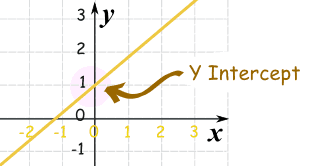Standard

# SLOPE = m

## Steps to calculate slope:

1. In order to calculate slope you need……. 2 PERFECT POINTS.  Write them down.
2. Once you have the 2 points/ordered pairs you can find the slope formula on the…… STAAR CHART. Write the formula down.
3. Now you MUST LABEL your 2 perfect points. ***Remember it is (x, y) the x value is 1st
4. Now that your points are labeled you can PLUG IN or substitute your numbers in the formula.
5. You can solve using your…… CALCULATOR.
6. If you get a decimal and want a fraction use your calculator and push the MATH button then ENTER and ENTER again and your answer should be in fractions form.

## Steps to calculate slope using only the graphing calculatorOther things you should know about SLOPE:                                                                                      Slope is a RATE, that means it happens again and again and again (multiple times, M). It is the ratio that explains how steep a line is. When you think of steepness think of a hill. The steeper the hill the harder it would be to walk up it. The harder to walk up the hill the greater the slope.

### Example: The RED line is steeper than the blue line. It would be harder to walk on. If you look at the  y=   equations on the right you can also see the slope of the RED line is greater than the slope of the BLUE line.– Love Ya’ll!!! Mrs. Molina 🙂

# Y-Intercept = b

## Steps to find the y-intercept:

1. Use your eyes and follow the line on the graph.
2. Notice where the lines touches or meets the y-axis ( the one that goes up and down)
3. The y value at this location is the y- intercept.

Example: The y-intercept (b) = 1# Unit Rate and Slope

Unit Rate and Slope are almost the EXACT SAME THING.

The ONLY difference is that Slope is just a number and Unit Rate must have a description with it.

Example:

# PYTHAGOREAN THEOREM

The Pythagorean Theorem states that, in a right triangle, the two smaller squares made from the triangles legs add up to equal the biggest square made from the hypotenuse.The lengths of the legs are commonly labeled as a and b and the hypotenuse length is labeled as c.REMEMBER:

HYPOTENUSE: c is the longest side of the triangle

LEGS: a and b are the other two sidesClick here  to find the missing hypotenuse , to find the missing leg, here  to find the perimeter, here  to do real world problems, and here to see if the triangle is a right triangle or not. You will use the Pythagorean Theorem online and get automatic feedback (it grades it)! 🙂

## What is the Pythagorean Theorem?

In short the Pythagorean Theorem can be explain with the formula below.

## a2 + b2 = c2

AGAIN, THIS IS ONLY TRUE FOR RIGHT TRIANGLES!

Here are other examples:Click here  to find the missing hypotenuse , to find the missing leg, here  to find the perimeter, here  to do real world problems, and here to see if the triangle is a right triangle or not. You will use the Pythagorean Theorem online and get automatic feedback (it grades it)! 🙂